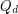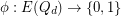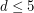Edge-antipodal colorings of cubes

 Importance: Medium ✭✭
 Author(s): Norine, Serguei
 Subject: Combinatorics » Ramsey Theory
 Keywords: antipodal cube edge-coloring
 Posted by: mdevos on: October 6th, 2008

We letdenote the-dimensional cube graph. A mapis called edge-antipodal ifwheneverare antipodal edges.

Conjecture   Ifandis edge-antipodal, then there exist a pair of antipodal verticeswhich are joined by a monochromatic path.

This conjecture has been verified by hand for.

Bibliography

* indicates original appearance(s) of problem.

2-colorings of edges of the cube

Q_n denotes the n-dimensional cube. For any x in Q_n, x_bar denotes the antipodal of x in Q_n.

We conjecture the following: Conj1 Let c:E_n --> {0, 1} be a coloring of the edges of Q_n. Then, there exists a pair of antipodal points x, x_bar and a path p from x to x_bar that it is either monochromatic or it changes colors exactly once.

It is easy to see that this conjecture implies an affirmative answer to the "antipodal" coloring open problem. We have verified that Conj1 holds for dimensions n=2, 3, and 4. We have also found that if the coloring is simple, that is, it does not contain squares colored 0101, then Conj1 holds (in fact, we find a monochromatic path joining a pair of antipodals).

proof?

Let's suppose G is minimal counterexample. We are denote vertices as "x1 x2 x3 ..." xi={0|1} so, for example, "110...01" and "001...10" are antipodes. Consider G' is subgraph induced by x1=1. G' is lesser hypercube, so where exists connected pair of G'-antipodes, for clarification, "100001111" and "111110000". but ("100001111","000001111") and ("111110000","011110000" ) are edge-antipodes in G, so either ("100001111", "011110000") or ("000001111", "111110000") are connected! (And path length is equal to G dimension.)

Looks too simple, am I misunderstood something? Or where is my medal? :))

not quite!

the edge-coloring of the subcube consisting of those vertices with x1=1 need not be edge-antipodal.

Yes, indeed

Got it. edge-antipodes in G' are not antipodes in G, so can have same color. Thanks.

special case proven

We prove the conjecture in the special case where there is no square xyzt in the cube such that xy and zt get value 0, while yz and xt get value 1. The paper by Tomas Feder and Carlos Subi (submitted) can be found at

http://theory.stanford.edu/~tomas/antipod.ps